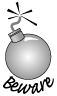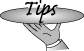### 6.5. if 语句

An if statement conditionally executes another statement based on whether a specified expression is true. There are two forms of the if: one with an else branch and one without. The syntactic form of the plain if is the following:

if 语句根据特定表达式是否为真来有条件地执行另一个语句。if 语句有两种形式：其中一种带 else 分支而另一种则没有。根据语法结构，最简单的 if 语句是这样的：

```     if (condition)
statement
```

The condition must be enclosed in parentheses. It can be an expression, such as

```     if (a + b > c) {/* ... */}
```

or an initialized declaration, such as

```     // ival only accessible within the if statement
if (int ival = compute_value()) {/* ... */}
```

As usual, statement could be a compound statementthat is, a block of statements enclosed in curly braces.

When a condition defines a variable, the variable must be initialized. The value of the initialized variable is converted to bool (Section 5.12.3, p. 181) and the resulting bool determines the value of the condition. The variable can be of any type that can be converted to bool, which means it can be an arithmetic or pointer type. As we'll see in Chapter 14, whether a class type can be used in a condition depends on the class. Of the types we've used so far, the IO types can be used in a condition, but the vector and string types may not be used as a condition.

To illustrate the use of the if statement, we'll find the smallest value in a vector<int>, keeping a count of how many times that minimum value occurs. To solve this problem, we'll need two if statements: one to determine whether we have a new minimum and the other to increment a count of the number of occurrences of the current minimum value:

```     if (minVal > ivec[i])  { /* process new minVal */ }
if (minVal == ivec[i]) { /* increment occurrence count */ }
```

#### 语句块用作 if 语句的对象

We'll start by considering each if in isolation. One of these if statements will determine whether there is a new minimum and, if so, reset the counter and update minVal:

```     if (minVal > ivec[i]) { // execute both statements if condition is true
minVal = ivec[i];
occurs = 1;
}
```

The other conditionally updates the counter. This if needs only one statement, so it need not be enclosed in curlies:

```     if (minVal == ivec[i])
++occurs;
```It is a somewhat common error to forget the curly braces when multiple statements must be executed as a single statement. 当多个语句必须作为单个语句执行时，比较常见的错误是漏掉了花括号。

In the following program, contrary to the indentation and intention of the programmer, the assignment to occurs is not part of the if statement:

```     // error: missing curly brackets to make a block!
if (minVal > ivec[i])
minVal = ivec[i];
occurs = 1; // executed unconditionally: not part of the if
```

Written this way, the assignment to occurs will be executed unconditionally. Uncovering this kind of error can be very difficult because the text of the program looks correct.Many editors and development environments have tools to automatically indent source code to match its structure. It is a good idea to use such tools if they are available. 很多编辑器和开发环境都是提供工具自动根据语句结构缩排源代码。有效地利用这些工具将是一种很好的编程方法。

#### 6.5.1. if 语句的 else 分支

Our next task is to put these if statements together into an execution sequence. The order of the if statements is significant. If we use the following order

```     if (minVal > ivec[i]) {
minVal = ivec[i];
occurs = 1;
}
// potential error if minVal has just been set to ivec[i]
if (minVal == ivec[i])
++occurs;
```

our count will always be off by 1. This code double-counts the first occurrence of the minimum.

Not only is the execution of both if statements on the same value potentially dangerous, it is also unnecessary. The same element cannot be both less than minVal and equal to it. If one condition is true, the other condition can be safely ignored. The if statement allows for this kind of either-or condition by providing an else clause.

The syntactic form of the if else statement is

if else 语句的语法形式为：

```     if (condition)
statement1
else
statement2
```

If condition is true, then statement1 is executed; otherwise, statement2 is executed:

```     if (minVal == ivec[i])
++occurs;
else if (minVal > ivec[i]) {
minVal = ivec[i];
occurs = 1;
}
```

It is worth noting that statement2 can be any statement or a block of statements enclosed in curly braces. In this example, statement2 is itself an if statement.

##### 悬垂 else

There is one important complexity in using if statements that we have not yet covered. Notice that neither if directly handles the case where the current element is greater than minVal. Logically, ignoring these elements is finethere is nothing to do if the element is greater than the minimum we've found so far. However, it is often the case that an if needs to do something on all three cases: Unique steps may be required if one value is greater than, less than, or equal to some other value. We've rewritten our loop to explicitly handle all three cases:

```     // note: indented to make clear how the else branches align with the corresponding if
if (minVal < ivec[i])
{ }                       // empty block
else if (minVal == ivec[i])
++occurs;
else {                        // minVal > ivec[i]
minVal = ivec[i];
occurs = 1;
}
```

This three-way test handles each case correctly. However, a simple rewrite that collapses the first two tests into a single, nested if runs into problems:

```     // oops: incorrect rewrite: This code won't work!
if (minVal <= ivec[i])
if (minVal == ivec[i])
++occurs;
else {      // this else goes with the inner if, not the outer one!
minVal = ivec[i];
occurs = 1;
}
```This version illustrates a source of potential ambiguity common to if statements in all languages. The problem, usually referred to as the dangling-else problem, occurs when a statement contains more if clauses than else clauses. The question then arises: To which if does each else clause belong? 这个版本表明了所有语言的 if 语句都普通存在着潜在的二义性。这种情况往往称为悬垂 else 问题，产生于一个语句包含的 if 子句多于 else 子句时：对于每一个 else，究竟它们归属哪个 if 语句？

The indentation in our code indicates the expectation that the else should match up with the outer if clause. In C++, however, the dangling-else ambiguity is resolved by matching the else with the last occurring unmatched if. In this case, the actual evaluation of the if else statement is as follows:

```     // oops: still wrong, but now the indentation matches execution path
if (minVal <= ivec[i])
// indented to match handling of dangling-else
if (minVal == ivec[i])
++occurs;
else {
minVal = ivec[i];
occurs = 1;
}
```

We can force an else to match an outer if by enclosing the inner if in a compound statement:

```     if (minVal <= ivec[i]) {
if (minVal == ivec[i])
++occurs;
} else {
minVal = ivec[i];
occurs = 1;
}
```Some coding styles recommend always using braces after any if. Doing so avoids any possible confusion and error in later modifications of the code. At a minimum, it is nearly always a good idea to use braces after an if (or while) when the statement in the body is anything other than a simple expression statement, such as an assignment or output expression. 有些编程风格建议总是在 if 后面使用花括号。这样做可以避免日后修改代码时产生混乱和错误。至少，无论 if（或者 while）后面是简单语句，例如赋值和输出语句，还是其他任意语句，使用花括号都是一个比较好的做法。

## Exercises Section 6.5.1

 Exercise 6.5: Correct each of the following: 改正下列代码： ``` (a) if (ival1 != ival2) ival1 = ival2 else ival1 = ival2 = 0; (b) if (ival < minval) minval = ival; // remember new minimum occurs = 1; // reset occurrence counter (c) if (int ival = get_value()) cout << "ival = " << ival << endl; if (!ival) cout << "ival = 0\n"; (d) if (ival = 0) ival = get_value(); ``` Exercise 6.6: What is a "dangling else"? How are else clauses resolved in C++? 什么是“悬垂 else”？C++ 是如何匹配 else 子句的？